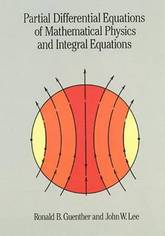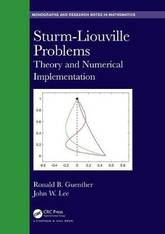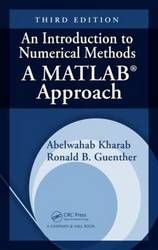#### Ronald B. Guenther author

Image credit: dr. shordzi, on Flickr
Filter
(found 5 products)Partial Differential Equations of Mathematical Physics and Integral Equations
30.400000 USD

## Partial Differential Equations of Mathematical Physics and Integral Equations

by John W. Lee, Ronald B. Guenther
Paperback / softbackSturm-Liouville problems arise naturally in solving technical problems in engineering, physics, and more recently in biology and the social sciences. These problems lead to eigenvalue problems for ordinary and partial differential equations. Sturm-Liouville Problems: Theory and Numerical Implementation addresses, in a unified way, the key issues that must be faced ...
Sturm-Liouville Problems: Theory and Numerical Implementation
Sturm-Liouville problems arise naturally in solving technical problems in engineering, physics, and more recently in biology and the social sciences. These problems lead to eigenvalue problems for ordinary and partial differential equations. Sturm-Liouville Problems: Theory and Numerical Implementation addresses, in a unified way, the key issues that must be faced in science and engineering applications when separation of variables, variational methods, or other considerations lead to Sturm-Liouville eigenvalue problems and boundary value problems.
251.06 USD

## Sturm-Liouville Problems: Theory and Numerical Implementation

by John W. Lee, Ronald B. Guenther
HardbackHighly recommended by CHOICE, previous editions of this popular textbook offered an accessible and practical introduction to numerical analysis. An Introduction to Numerical Methods: A MATLAB (R) Approach, Third Edition continues to present a wide range of useful and important algorithms for scientific and engineering applications. The authors use MATLAB ...
An Introduction to Numerical Methods: A MATLAB Approach, Third Edition
Highly recommended by CHOICE, previous editions of this popular textbook offered an accessible and practical introduction to numerical analysis. An Introduction to Numerical Methods: A MATLAB (R) Approach, Third Edition continues to present a wide range of useful and important algorithms for scientific and engineering applications. The authors use MATLAB to illustrate each numerical method, providing full details of the computer results so that the main steps are easily visualized and interpreted. New to the Third Edition A chapter on the numerical solution of integral equations A section on nonlinear partial differential equations (PDEs) in the last chapter Inclusion of MATLAB GUIs throughout the text The book begins with simple theoretical and computational topics, including computer floating point arithmetic, errors, interval arithmetic, and the root of equations. After presenting direct and iterative methods for solving systems of linear equations, the authors discuss interpolation, spline functions, concepts of least-squares data fitting, and numerical optimization. They then focus on numerical differentiation and efficient integration techniques as well as a variety of numerical techniques for solving linear integral equations, ordinary differential equations, and boundary-value problems. The book concludes with numerical techniques for computing the eigenvalues and eigenvectors of a matrix and for solving PDEs. CD-ROM Resource The accompanying CD-ROM contains simple MATLAB functions that help students understand how the methods work. These functions provide a clear, step-by-step explanation of the mechanism behind the algorithm of each numerical method and guide students through the calculations necessary to understand the algorithm. Written in an easy-to-follow, simple style, this text improves students' ability to master the theoretical and practical elements of the methods. Through this book, they will be able to solve many numerical problems using MATLAB.